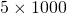# Estimating (Multiplication)

When we don’t need a precise answer, it is helpful to confidently estimate within a good error margin. For example, if buying lumber, you need to buy 42 feet at 5.83 per foot, what is the ball park for the price?

One method is to round each value to one significant figure:

42 simplifies to 40

5.83 simplifies to 6

Now lets take.

Now, using a calculator we find that.

We underestimated, but not by very much. As a percentage of the precise answer, our estimate is out by 1.98%.

In this context of practising arithmetic, a good estimate is within 10% of the precise answer. An excellent estimate is within 5% of the precise answer.

Another example:.

Now, 422 simplifies to 400, or.

5123 simplifies to 5000, or.

So we haveUsing a calculator, we find that.

Our error is 7.49%.

Try it out!

## A little more difficult

Done here – go back to the Numeracy Menu